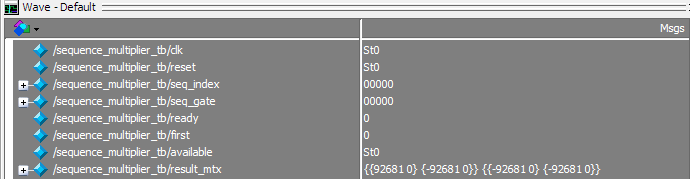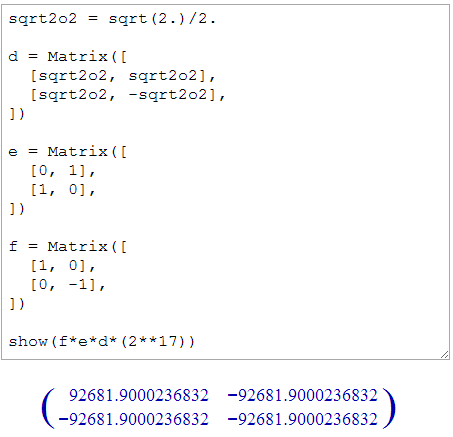# Matrix Sequence Multiplier Finished!

This week, I implemented the sequence multiplier, a module that multiplies a sequence of 2×2 matrices together and provides the result.  Implementing this module involved:

1. Completing and testing the gate table module from last week, which provides the matrix for a gate given that gate’s index number.
2. Adjusting the fixed-point number format to use the most significant bit to store a one.

Fixed Point Format Adjustments

I’m using a fixed-point format to represent numbers in matrix calculations, since one property of these calculations is that the magnitude of the numbers won’t change significantly.  My original design used all available bits for the fractional part of the number, but this means a one can’t be stored.  Thus, I would essentially have to use .99999 instead of 1, introducing unnecessary error.  More importantly, if a calculation results in a number slightly greater than 1, the 1 might be truncated, leaving only the fractional part.

So, I now use the most significant bit to store the one’s place digit.  This leaves 17 bits for the fractional part.  I can now represent numbers in the range (-2, 2).  This adjustment involved a minor change to the fixed-point multiplication module.

I might automatically truncating all matrix cells to be within the range [-1, 1], since no matrix member should exceed this range.  This could eliminate some error, but may not be worth the additional logic delay.

Simulating the Sequence Multiplier

Here’s a screenshot of the ModelSim output.  result_mtx is a bus that contains the result of the matrix multiplication.  The contents are grouped with braces in row-major order, each complex number is displayed in the format {real-part, imaginary-part}.I verified this result using Sage, a free computer algebra system available at sagenb.org.  I multiplied the first three gates together.  Then, I multiplied the result by 2^17, because 17 of the 18 bits in my fixed-point numbers are part of the fraction.  Thus, the result I get reflects what I would see in simulation:The results are only off by one, if you consider the lack of rounding.  This precision is not common, though: the first two matrices involved multiplication by one.

Coming Up This Weekend/Next Week

My main goal is to get performance numbers for the algorithm (1) on the computer, and (2) on the board.  In later weeks, I’ll apply optimizations and analysis to each implementation, to see how much the performance can improve.

• Implement the brute-force sequence generator. If you imagine that each gate is a digit in a number, this generator is nothing more than an incrementor.
• Implement a brute-force version of the algorithm. This part involves little more than connecting up modules I’ve already developed.  It will not check for duplicates, because I haven’t gained access to the onboard RAM yet.
• Implement communication between the board and the computer over the serial port.  The computer will provide a gate to compile, and the board will report the result when found.  It will also periodically indicate progress and performance statistics.
• Increase numeric precision to 36 bits. This process is fairly straightforward, but it may complicate the process of fitting things onto the board.
• Download the design onto the board. This won’t happen until Monday evening at the earliest.  This step involves getting the design to fit, meaning I need to intelligently schedule the hardware multipliers.
• Start drafting the paper.

If you want to read my current paper draft, look at my source code on GitHub, etc., click Quantum Compiler at the top of this page.

This entry was posted in Quantum Compiler Research. Bookmark the permalink.